# SP12. Solutions to

### SP12. Solutions to selected problems

#### Problem SP1.1.

One difference between water and these other molecules is that water is polar: there is a significant electronegativity difference between the oxygen and the hydrogen. The charges in one water molecule may be interacting with charges in other water molecules.

#### Problem SP3.1.

As far as we can tell from these data, the melting point of nitrogen and oxygen would be somewhere around -220°C, whereas the boiling point would be around -180°C. We would expect these numbers to be similar to fluorine, which has a similar mass. In fact, the melting and boiling points of oxygen are about -219°C and -183°C, respectively. The melting and boiling points of nitrogen are about -210°C and -196°C, respectively.

#### Problem SP4.1.

1. A chain that is 8 carbons long would have much lower London interactions than a chain that is 102 atoms long. The greater the contact between molecules, the greater the chance for weak attractions due to small charges arising from random electron movement. The molecule with the longer chain would have the higher melting point, because more energy would have to be added in order to overcome those attractions and get the molecules moving.
2. The first chain is more linear whereas the second chain is more branched. The more linear molecules will pack together more easily, allowing greater surface contact for increased London interactions. The straighter chain would have a higher melting point.
3. Bromomethane has a lot more electrons than chloromethane. Based on London interactions alone, we would expect bromomethane to have a higher melting point. In fact, the melting point of bromomethane is about -94°C, compared to about -97°C for chloromethane; there must be another factor that compensates for the difference in numbers of electrons and makes these values so close.

#### Problem SP4.2.

Because of the large numbers of electrons in bromine, we would expect a higher boiling point in 3,4-dibromohexane.

#### Problem SP4.3.

Remember benzyl refers to the group C6H5CH2 (below right); cyclohexylmethyl suggests the superficially similar C6H12CH2 (below left).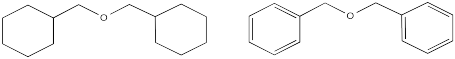The benzyl group contains trigonal planar carbons, whereas the cyclohexylmethyl group contains only tetrahedral carbons. The benzyl groups,being flatter, could pack more easily together and interact more strongly. We would expect dibenzyl ether to have a higher melting point.

#### Problem SP5.1.

These compounds all contains lots of non-polar C-C and C-H bonds, which would not have appreciable dipoles. However, in each pair, there is a compound that contains more polar bonds as well: C-Cl, C-N and C-O.

#### Problem SP5.2.

Dipoles are vectors. If we have two polar bonds, they will add together using vector addition. Any vector can be shown as the sum of two other vectors. Below, the red arrow points down and to the left. How far down and how far to the left? We can show how far using the blue arrows. The blue arrow down and the blue arrow to the left add up to give the red arrow that goes down and to the left.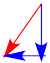In dichloromethane, there are two polar bonds. Both bonds are polarized towards the more electronegative chlorine. As drawn below, one bond has a dipole straight to the right. The other bond has a dipole that goes down and to the left. If the molecule has an overall dipole, with a positive end of the whole molecule and a negative end of the whole molecule, which end would be positive and which would be negative?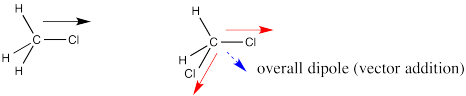It seems reasonable that the positive end would be somewhere over on the hydrogen side and the negative end would be somewhere over on the chlorine side. The overall dipole, as opposed to the individual bond dipoles, would point somewhere in the direction of the blue, dashed arrow. But how big would it be? Surely it's bigger than either of the two individual dipoles, because they are adding together, right?

Let's take a very qualitative look. The first red arrow is just to the right. We'll break the second arrow into a smaller arrow down and an even smaller arrow to the left. The sum of the left/right arrows is a very small arrow to the right.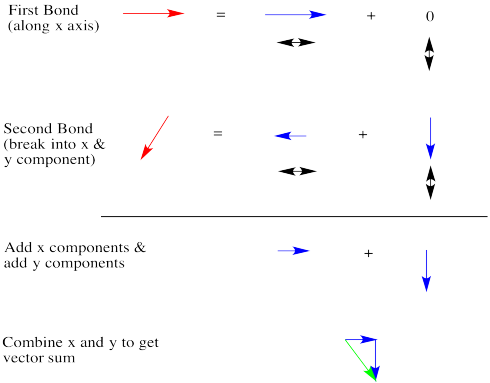The sum of the up/down arrows is just a small arrow down. Overall, the sum is a green arrow going down and to the right. The result doesn't really seem any bigger than an individual bond dipole.

#### Problem SP5.3.

Despite the relatively similar dipole moments, dichloromethane's boiling point is much higher than chloromethane's. The difference probably lies in the much greater mass of dichloromethane.

#### Problem SP5.4.

In each case, the one on the right has a larger dipole and a higher melting point.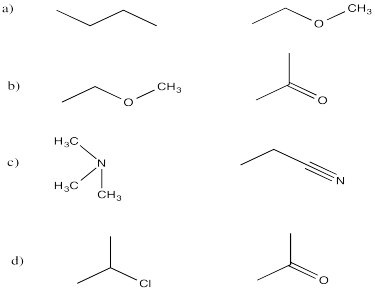#### Problem SP6.1.

Only ethylamine and ethanoic acid have the N-H or O-H bonds that are polar enough for hydrogen bonding.

#### Problem SP6.2.

hexylamine > dipropylamine > triethylamine

The one with the greatest potential for hydrogen bonding will have the highest melting point.

#### Problem SP7.1.

a) 2-propenol b) heptanoic acid c) 1-hexen-3-one

#### Problem SP8.1.

Hydrogen bonding.

#### Problem SP8.2.

a) dipole interactions b) London interactions c) dipole interactions d) hydrogen bonding

#### Problem SP8.3.

Water molecules interact with each other mainly through hydrogen bonding, whereas octane molecules interact with each other via London interactions. The main problem here is the strong hydrogen bonding between the water molecules. All molecules, in principle, could interact with each other via London interactions. However, if octane molecules were introduced among the water molecules, they would take up space. Some of the water molecules would not be able to get close enough to each other to hydrogen bond anymore. The loss of that very stabilizing interaction would be too costly.

#### Problem SP8.4.

This problem is similar to the previous one, but in this case the attraction between the strong dipoles of the nitrile groups would be too much to overcome.

#### Problem SP8.5.

a) dipole / induced dipole b) dipole / dipole c) hydrogen bonding d) dipole / induced dipole

#### Problem SP9.1.

The predicted order, from most soluble to least, would be LiCl > MgSO4 > AlPO4 because the ions increase in charge from 1+/- to 2+/- to 3+/-

#### Problem SP9.2.

The predicted order, from most soluble to least, would be dimethylsulfoxide > acetonitrile > pyridine > dichloromethane > triethylamine.

#### Problem SP10.1.

a) H-bond acceptor b) H-bond acceptor c) fully H-bonding d) H-bond acceptor e) fully H-bonding f) neither